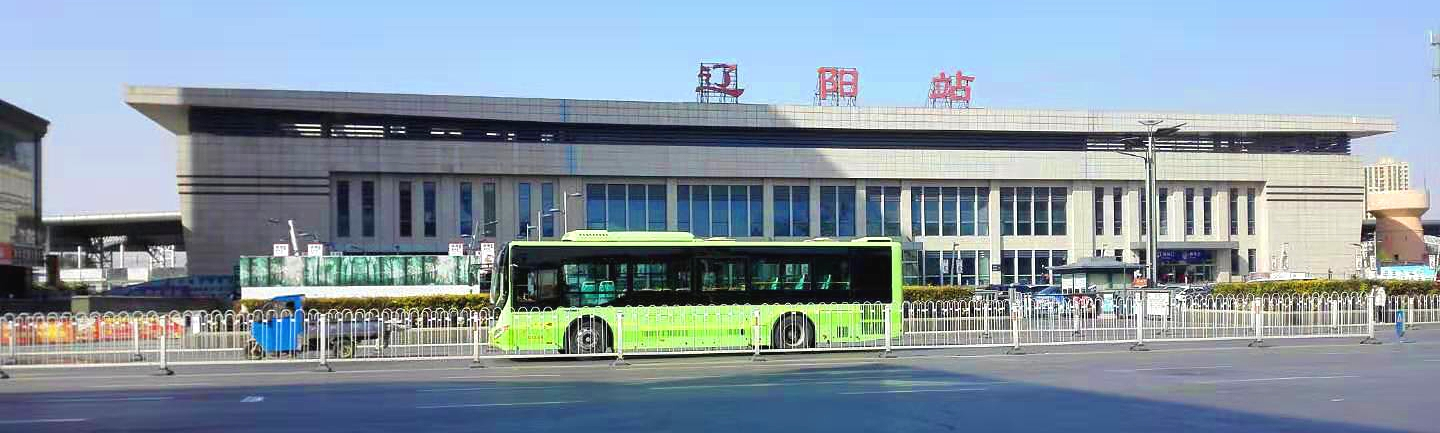公交线路信息1： 　　　　　市公汽公司线路001路=01站点周边　   　　   002路=02站点周边003路=03站点周边　   　　   004路西环=04站点周边005路=05站点周边　   　　   006路=06站点周边007路=07站点周边　   　　   008路直线=08站点周边009路=09站点周边　   　　   010路=10站点周边011路=11站点周边　   　　   012路=12站点周边013路=13站点周边　   　　   014路=14站点周边015路=15站点周边　   　   　016路=16站点周边017路=17站点周边　   　   　018路=18站点周边019路正线=19站点周边   　　020路=20站点周边021路=21站点周边　   　    　022路=22站点周边023路=23站点周边　   　    　024路=24站点周边025路=25站点周边　   　    　026路=26站点周边027路西环=27站点周边　    　028路=28站点周边029路=29站点周边　   　    　030路=30站点周边031路夏季=31站点周边　　　032路=32站点周边033路南环=33站点周边　    　035路=35站点周边036路=36站点周边　   　     　037路=37站点周边039路=39站点周边　   　     　040路=40站点周边041路=41站点周边　   　     　042路=42站点周边043路=43站点周边　   　     　049路=49站点周边050路=50站点周边　   　     　053路=53站点周边059路(学)=59站点周边　    　 060路(学)=60站点周边 公交线路信息2： 　　　　　　　公交化线路101路=a1站点周边　   　    　102路=a2站点周边103路正线=a3站点周边　   　104路窑地=a4站点周边104路=a4（站点+10）周边　108路正线=a8站点周边　　　　 　辽阳县公交线路201路=b01站点周边　   　   　202路=b02站点周边203路=b03站点吧　   　      　204路=b04站点周边205路=b05站点周边　   　      206环路=b06站点周边207路=b07站点周边　         　209路=b09站点周边210路=b10站点周边　         　211路=b11站点周边212路=b12站点周边　   　      213路区间=b13站点周边　　　　　 灯塔公交线路301路=c1站点周边　   　　　 302路=c2站点周边303路=c3站点周边　   　　　 304路=c4站点周边305路=c5站点周边 公交线路信息3： 公交化线路---辽阳境内客运线路（部分）辽阳-弓长岭=k01站点周边辽阳-小北河=k02站点周边辽阳-灯塔(东道)=k03站点周边辽阳-灯塔（直达）=k04站点周边辽阳-灯塔(西道)=k04（站点+10）周边灯塔-佟二堡=k05站点周边辽阳-佟二堡=k05（站点+10）周边辽阳-刘二堡=k06站点周边辽阳-岳家=k07站点周边宏伟外环(东环)=k08站点周边忠旺北门-旭家=k09站点周边辽化华润万家-洋湖沟=k10站点周边注明：本网站信息系统编码规则：等号前边是线路信息，等号后边是线路站点周边信息的编码。1.市公交线路信息采用两位数字，2.站点信息采用两位数字，3.周边门店等信息采用两位数字，4.非市公汽公司线路的三位数线路   百位数改用对应字母1=a、2=b、3=c   如：101=a1、201=b01、301=c1，5.境内客运线路用k字母加以标注并说明。这样编码后有利于本网站对信息的管理。

2020-01-16
2020-02-01
2020-01-30
2020-01-28
2020-01-23